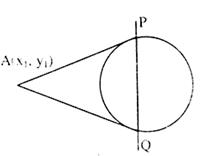# Ice cream on the side

Calculus Level 4For a circle $\displaystyle x^{2}+y^{2}=r^{2}$ ,find the value of r for which the area enclosed by the tangents drawn from the point $\displaystyle P(6,8)$ to the circle and the chord of contact is maximum.

×